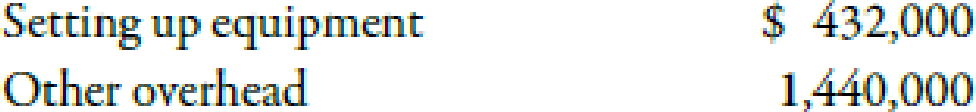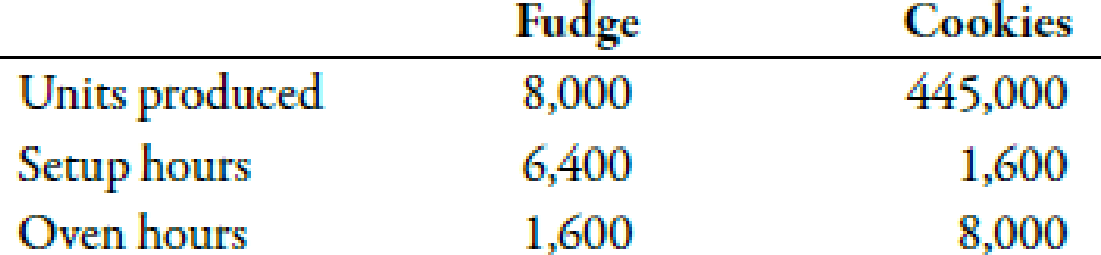Chapter 5, Problem 39E### Managerial Accounting: The Corners...

7th Edition
Maryanne M. Mowen + 2 others
ISBN: 9781337115773

#### Solutions

Chapter
Section### Managerial Accounting: The Corners...

7th Edition
Maryanne M. Mowen + 2 others
ISBN: 9781337115773
Textbook Problem
856 views

# Comparing ABC and Plantwide Overhead Cost AssignmentsWellington Chocolate Company uses activity-based costing (ABC). The controller identified two activities and their budgeted costs:Setting up equipment is based on setup hours, and other overhead is based on oven hours.Wellington produces two products, Fudge and Cookies. Information on each product is as follows:Required:(Note: Round answers to two decimal places.) 1. Calculate the activity rate for (a) setting up equipment and (b) other overhead. 2. How much total overhead is assigned to Fudge using ABC? 3. What is the unit overhead assigned to Fudge using ABC? 4. Now, ignoring the ABC results, calculate the plantwide overhead rate, based on oven hours. 5. How much total overhead is assigned to Fudge using the plantwide overhead rate? 6. CONCEPTUAL CONNECTION Explain why the total overhead assigned to Fudge is different under the ABC system (i.e., using the activity rates) than under the non-ABC system (i.e., using the plantwide rate).

1.

To determine

Compute the activity rate for setting equipment and other overhead.

Explanation

Activity Based Costing (ABC):

Activity based costing is an apportionment of costs that first considers the activity drivers that helps in the allocation of costs to various activities and then allocates costs to different cost objects by using the drivers.

a.

Use the following formula to calculate activity rate for setting equipment:

Activity rate=Set-up costsTotal set-up hours

Substitute $432,000 for set up costs and$8,000 for set up costs in the above formulae.

Activity rate=$432,000$8,000=$54per set up hour Therefore, the activity rate of setting equipment is$54 per set up hour.

b

2.

To determine

Calculate the total overhead cost which is assigned to fudge with the help of ABC approach.

3.

To determine

Calculate the unit overhead cost which is assigned to fudge with the help of ABC approach.

4.

To determine

Compute the plant wide overhead rate by using oven hours.

5.

To determine

Compute the total overhead costs which are assigned to fudge with the help of plant wide overhead rate.

6.

To determine

Discuss the reason for difference in the values of total overhead by using ABC system and by using plant wide overhead rate.

### Still sussing out bartleby?

Check out a sample textbook solution.

See a sample solution

#### The Solution to Your Study Problems

Bartleby provides explanations to thousands of textbook problems written by our experts, many with advanced degrees!

Get Started